Mobile QR Code1. (Department of Materials Science and Engineering, Hongik University, 94 Wausan-ro, Mapo-gu, Seoul, 04066, Korea)

Neuromorphic computing, phasechange, synapse device, device simulation, thermal analysis

## I. INTRODUCTION

Neuromorphic computing recently receives a lot of attention as a hardware counterpart of artificial intelligence (AI) system to a software AI technology that is performed on conventional von-Neumann architecture composed of separate logic and memory chips, for instances, central-processing-unit, main memory, and auxiliary memory or storage. The von-Neumann architecture has problems in power consumption, data transfer, system size when neural network (NN) algorithms using large-scale data such as deep neural network are performed. On the other hand, the neuromorphic architecture is composed of neurons and synapse devices as a biological brain does. This new system is optimal for performing NNs when energy consumption and system size are considered[1,2].

In a neuromorphic chip, neuron devices integrate input signals from multiple dendrites and fire a signal when the summation of inputs exceed a threshold value. Synapse devices contain information on the connection strength between neurons. The connection strength is more frequently called synaptic weight. The synaptic weight is gradually strengthened or weakened to the spike-timing between pre- and post-synaptic neurons, which is called spike-timing-dependent plasticity (STDP). The fully weakened state can be regarded as zero while the fully strengthened state can be regarded as one. There are many intermediate states in the weight. Storing the weight information requires many bits if a conventional on/off (or binary) memory is used. However, if a memory with many intermediate states is used, the synaptic weight can be stored in just a single bit, which saves a lot of power and space in the system. The phase-change random access memory (PC-RAM) is a strong candidate for the synapse device because it can have many intermediate resistances. Many research activities have been conducted for fabricating synapses by utilizing the PC-RAM technology[3-8].

The most common active material for the PC-RAM is Ge2Sb2Te5 (hereafter, GST) that can change reversibly between amorphous and crystalline states by applying an appropriate electrical pulse. The amorphous state with high resistivity is made by a melt-quench process corresponding to a short high-powered pulse (10~50 ns) while the crystalline state with low resistivity is made by annealing corresponding to a long moderate-powered pulse (50~200 ns). In the operation temperature range (less than 85°C), both states are stable enough to guarantee ten-year data retention.

Heat plays a key role in both phase-changes - amorphization and crystallization. The heat is generated by Joule heating and the generated heat flows by conduction within a solid-state device. These heat phenomena are controlled by the device architecture including device geometry, materials, and interfaces between the materials. Among these, thermal boundary resistance (TBR) between the GST and other material interferes the flow of the heat generated in the GST region. In other words, the TBR acts as a heat retainer and contributes to reducing the reset current, that is, the minimum current value that can melt the GST. In this reason, the cell architecture of the PC-RAM has evolved from the line-and-space type to the confined type. The cell types were named after the layout of the storage node (the GST) as shown in Fig. 1. The confined type has four-sided TBRs encompassing the GST while the line-and-space type has two-sided TBRs. Hence, the confined type has lower reset current and less thermal interference to adjacent cells than the line-and-space type does, which is very critical for memory application. In addition, the confined cell has abrupt transition from low resistance state (LRS) to high resistance state (HRS), which also means that heat is efficiently utilized due to the four-sided TBRs. However, in case of synapse application, the abrupt transition is problematic for storing gradual synaptic weight, which can be understood in terms of sensitivity or controllability. It is hard to obtain a precisely controlled intermediate state in an abrupt transition region.

Fig. 1. Schematic diagram of the confined and the line-and-space architectures and the layouts of the active material.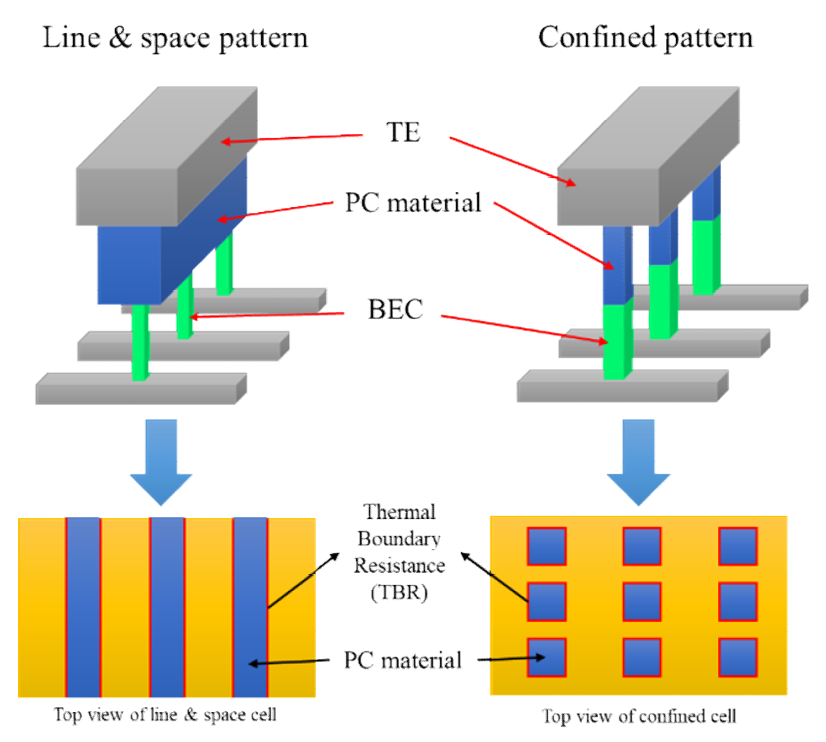In this paper, our investigation on the cell architecture for synapses using a TCAD simulation will be presented. Our goal is finding a cell architecture that realizes more gradual transition from LRS to HRS, in other words, a wider transition region in a current-resistance curve. Initially, our focus was on the cutting-edge confined architecture because it is the current industry trend in the sub 20 nm PC-RAM development. It was found that a confined GST cell with higher aspect ratio showed slightly less abrupt transition. Then, we came up with the line-and-space GST with infinite aspect ratio that in turn showed much more gradual transition. Details on our results will be described in the next sections.

## II. MODELING OF SWITCHING OPERATIONS IN PC-RAM

Simulating PC-RAMs requires the integrated calculation of electrical, thermal, and phase-change models. Reset and set operations of a PC-RAM cell can be simulated using the Sentaurus TCAD simulator version L-2016.03 that contains a phase-change module. At a time-step, the device simulator numerically solves drift-diffusion equations to obtain the current density distribution within the device for applied bias conditions. Either of voltage and current sources can be selected. Then it calculates Joule heating and heat dissipation in order to obtain temperature distribution by solving the heat equation with a heat source term. Finally, it calculates phase-change at each mesh point in the GST region using phase-change equations that will be explained later in this section. All these calculations are self-consistent and are iteratively performed at each step using the information from the previous time step.

The phase-change kinetics equations are less commonly known compared to the D-D equations and the heat equation. Therefore, we would like to provide more explanation on it. There exist three phases (crystalline, amorphous, and melt) and six transitions between the phases in the GST. However, two transitions, the crystal-to-amorphous and the melt-to-crystal transitions, are thermodynamically impossible. The crystalline phase always has lower free energy than the amorphous phase. A crystal cannot directly become amorphous without passing through melt except some very special cases, for example, a damaged silicon substrate by ion implantation. The melt-to-crystal transition can occur if the melt is slowly cooled down. However, this is in fact the melt-to-amorphous-to-crystal transition in such a way that in the cooling process the melt initially becomes amorphous at $T_{m}$ and then the amorphous crystallizes during further slow cooling. If the amorphous is rapidly cooled (quenched), the amorphous remains at room temperature. With these reasons, we exclude the two transitions. In addition, it can be assumed that melting speed is the same for both crystal and amorphous. Hence, only three kinetics such as melting, quenching, and crystallization need be considered.

The phase fractions are expressed with three phase variables, $S_{c}(\vec{r})$, $S_{a}(\vec{r})$, and $S_{m}(\vec{r})$ whose subscripts denote crystalline, amorphous, and melt. All the phase variables have a value between zero and one. At a position $\vec{r}$ , the summation of the phase variables must be one. A position with $S_{c}$ = 0.9, $S_{a}$ = 0.1, and $S_{m}$ = 0 have 90% crystalline phase and 10% amorphous phase. In this way, the phase distribution within the GST region can be well described as a function of position. The melting is just determined by a rapid transition at the very moment when temperature reaches the melting temperature $T_{m}$. Note that above $T_{m}$ only the melt phase can exist. When the melt phase is cooled down, the melt primarily becomes amorphous, which is also described by a rapid transition (quenching), as explained before. Then the amorphous phase crystallizes if temperature remains below $T_{m}$. The crystallization kinetics depending upon temperature is not that simple, which is the most important part in the phase-change model. As a rule of thumb, the crystallization speed is very slow at low temperature and at high temperature close to $T_{m}$. In other words, a maximum crystallization speed appears at a temperature around 0.6-0.8 $T_{m}$ (absolute temperature scale) that is material-dependent.

The crystallization occurs via nucleation and growth process. Atoms in an amorphous phase move around constantly like "liquid" (but very slowly). At an instant, several atoms form a small crystalline cluster or a crystallite. Too small crystallites consist of fewer atoms disappears immediately because they are unstable due to the larger interface effect. Crystallites larger than a critical size start to grow, by which total free energy of the system decreases. The occurrence of a growing crystallite is at a rate, $I_{N}\left[\mathrm{cm}^{-3} \mathrm{s}^{-1}\right]$, that is called the nucleation rate. Each nucleus starts to grow at a speed, $V_{G}$ [cm/s], immediately after its formation. The macroscopic crystallization kinetics including all these effects is expressed by the Johnson-Mehl-Avrami-Kolmogorov equation that yields the "S-curve" for transformed fraction as a function of time, $X (t)$.

##### (1)
$X(t)=1-\exp \left(-b t^{n}\right)$

The parameters are $b=\pi V_{G}^{3} I_{N} / 3$ and n = 4 for an infinitely large 3D sample. This equation is valid for uniform temperature over the sample, which is not the case for the PC-RAM operations. Most importantly, the temperature dependent equations of the nucleation rate and the growth velocity are expressed as the followings[13,14].

##### (2)
$I_{N}=I_{N, 0} \exp \left[\frac{E_{a, N}+\Delta G^{*}(T)}{k T}\right]$

##### (3)
$V_{G}=V_{G, 0}\left[1-\exp \left(\frac{\Delta G^{*}(T)}{k T}\right)\right] \exp \left(\frac{E_{a, G}}{k T}\right)$

##### (4)
$\Delta G^{*}(T)=\frac{16 \pi}{3} \frac{\gamma^{3}}{\Delta G^{2}(T)}$

##### (5)
$\Delta G(T)=\left\{\begin{array}{ll}{\Delta \mathrm{H}_{C}\left[1-\frac{T}{T_{c}}\left(1-\frac{\Delta \mathrm{H}_{f}}{\Delta \mathrm{H}_{c}} \frac{T_{m}-T_{C}}{T_{m}}\right)\right]} & {\left(T < T_{c}\right)} \\ {\Delta \mathrm{H}_{f}\left(1-\frac{T}{T_{m}}\right)} & {\left(T_{c} < T < T_{m}\right)} \\ {0} & {\left(T > T_{m}\right)}\end{array}\right.$

The $I_{N, 0}$ and $I_{G, 0}$ are pre-exponential factors, $E_{a, N}$ and $E_{a, G}$ are activation energies, $\Delta G^{*}(T)$ is the nucleation barrier, $\gamma$ is the interface energy, $\Delta G(T)$ is the bulk free energy difference, $\Delta H_{c}$ and $\Delta H_{f}$ are the enthalpies of crystallization and fusion, and $T_{c}$ and $T_{m}$ are crystallization and melting temperatures. In the TCAD, the crystallization rate, ($\dot{s}_{c}\left(=-\dot{s}_{a}\right)$), below $T_{m}$ is described by the two contributions, the nucleation and the growth, by adopting Eq. (2), Eq. (3) as described in ref. 11.

##### (6)
$\dot{S}_{c}=r_{N, 0} \exp \left[\frac{E_{a, N}+\Delta G^{*}(T)}{k T}\right]$

##### (7)
$\dot{s}_{c}=r_{G, 0}\left[1-\exp \left(\frac{\Delta G^{*}(T)}{k T}\right)\right] \exp \left(\frac{E_{a, G}}{k T}\right)$

Eq. (6) corresponds to the nucleation while Eq. (7) does to the growth. The $r_{N, 0}$ and $r_{G, 0}$ are preexponential factors that need be calibrated. All other parameters that are all material dependent in Eq. (2)-Eq. (5) are also used in Eq. (6), Eq. (7). Simulation examples of the reset and the set operations for a simple PCM cell geometry are provided in Fig. 2 where all parameters were used as default in the simulator.

Fig. 2. Simulation examples of reset and set operations using Synopsys TCAD: the sample 2D geometry of a PC-RAM cell on the leftmost, pulse shapes in the middle, and simulated phase-fraction and temperature on the right.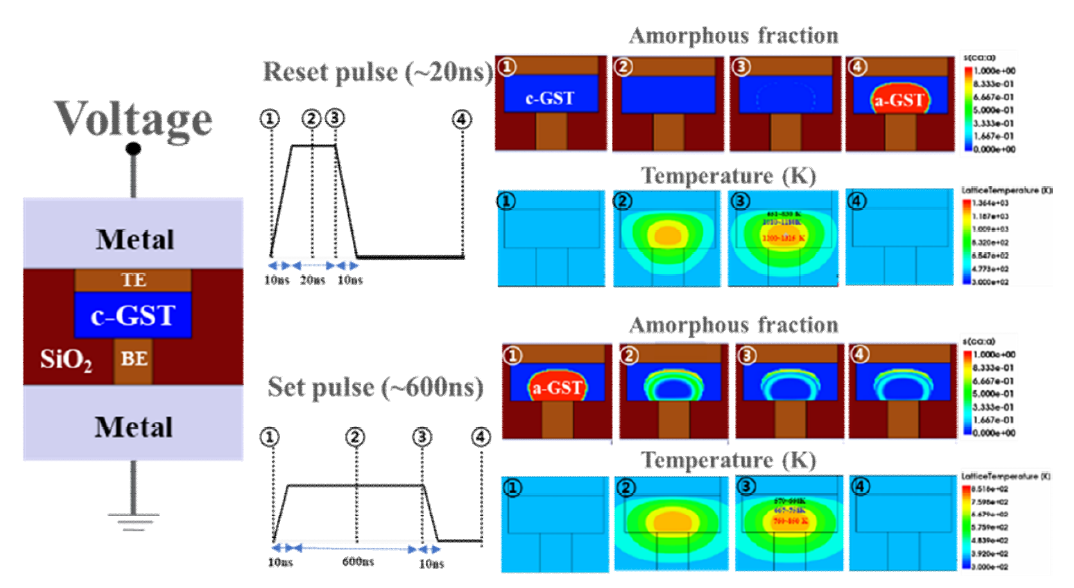## III. DATA AND RESULTS

### 1. GST Cell Structure Engineering

As mentioned in Section I, the characteristics of the PC-RAM are greatly affected by the geometry of its active material, GST [15, 16]. This is because the outflow of the heat generated within the GST region is greatly affected by the TBR at GST/other material interfaces[17-19]. Let us denote the GST and an adjacent material by 1 and 2, respectively. The flow of the heat leaving from the GST, $\vec{S}$ [W/cm2], is expressed as $\vec{S}=\left(T_{1}-T_{2}\right) / R_{t h}$ where $T_{1}$ and $T_{2}$ are temperatures [K] at material 1 and 2, and $R_{th}$ is the TBR [cm2·K/W]. The TBR values for GST/metal and GST/SiO2 interfaces were taken as 2.0 10-4 cm2·K/W and 7.5 10-4 cm2·K/W from ref. 18 and 19, respectively. In our simulations, temperature dependence was neglected because it is less than an order. These TBR values are comparable to the bulk thermal resistance of the GST itself, that is, the reciprocal of the thermal conductivity times the GST thickness. For the 10 nm-thick GST whose ends have 1 K temperature difference, its thermal resistance is (0.005 W/K·cm)-1 (10 10-7 cm) = 2.0 10-4 cm2K/W that is the same order with the TBR. Thus, both interface and bulk thermal resistances are important. All material and interface parameters used in our simulations are listed in Table 1[17-24]. Note that all metal parts in our simulation structures are tungsten. All values are taken from proper references.

Table 1. List of material properties applied in our TCAD simulation

 Parameter Value Unit Remark Resistivity c-GST 0.02 $\Omega$·cm [20, 21] a-GST 15.6  W 2.7 × 10-6  Thermal conductivity GST 0.005 W/cm·K  W 1.75  SiO2 0.014  Heat capacity GST 1.3 J/K·cm3  W 2.58  SiO2 3.1  Thermal boundary resistance GST/W 2.0 × 10-4 cm2·K/W  GST/SiO2 7.5 × 10-4 

We started with the confined GST patterns that are adopted for recent PC-RAMs. More specifically, they are the self-heating wall (SHW) and self-heating pillar (SHP) as shown in Fig. 3(a) and Fig. 3(b). On the right, there are the temperature profiles during the reset operation where a quarter of the whole structure is shown. The GST is confined meaning that there are four-sided TBRs in both x- and y-directions. They have the same height of h = 50 nm and the same cross-sectional area of A = 200 nm2. In case of the SHW, the width, W, and thickness, t, were varied maintaining the area. Two cases of (W = 20 nm, t = 10 nm) and (W = 25 nm, t = 8 nm) were simulated. In case of the SHP, the circular crosssection has the radius of 8 nm corresponding to the area of 200 nm2. Note that all cases have the same GST resistance and the only difference in these three cases is the lateral surface area through which the heat generated in the GST flows out. In other words, the larger lateral surface area yields the faster heat dissipation. The switching transition curves are presented in Fig. 3(c) where the GST cell with larger lateral surface area shows a little but clearly more gradual transition. This improvement is not enough at all although the clear trend is observed. The aspect ratio cannot be increased more than a certain limit because of the cell density.

Fig. 3. Simulation results for the confined patterns (a) self-heating wall (SHW) geometry and its temperature profile during reset, (b) self-heating pillar (SHP) geometry and its temperature profile during reset, (c) reset transition curves for three cases with the same resistance and different lateral surface areas.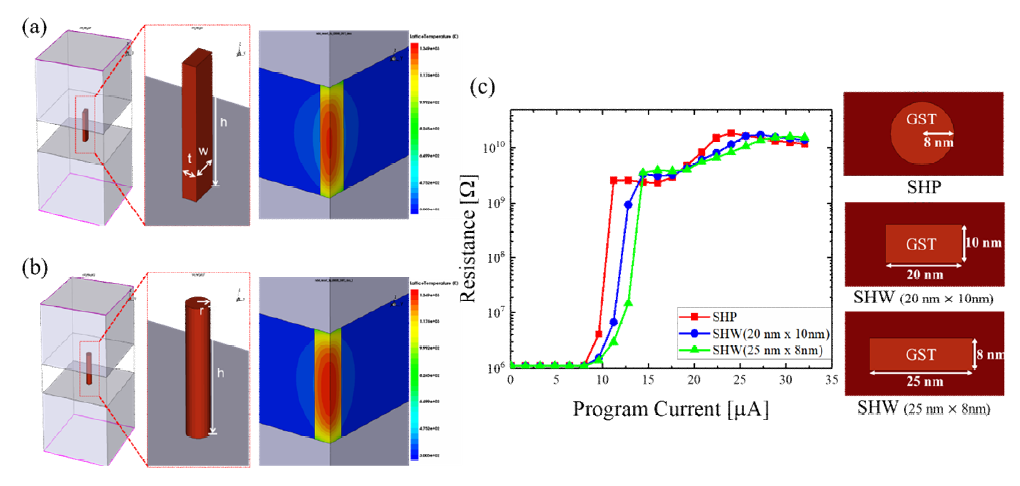At this point, we came up with the old-style line-andspace GST pattern that has inferior heat retaining ability because the GST has only two-sided TBRs. The heat flows away from the heat source toward the no-TBR directions. The simulation structure is shown in Fig. 4(a) where there is a temperature profile of a quarter structure during the reset operation. There are a line of GST and a wall-type heater underneath the GST. The height of the GST is h = 55 nm. The upper and the lower sides of the trapezoidal GST is 20 nm and 10 nm, respectively. Simulations were performed for three thickness values while keeping its width and height as 20 nm and 30 nm, respectively. The transition curves are presented in Fig. 4(b). The transition is clearly more gradual for a thicker heater through which more heat flows out through a thicker heater.

Fig. 4. Simulation results for the line-and-space pattern (a) the device geometry and its temperature profile during reset, (b) reset transition curves for different heater thicknesses.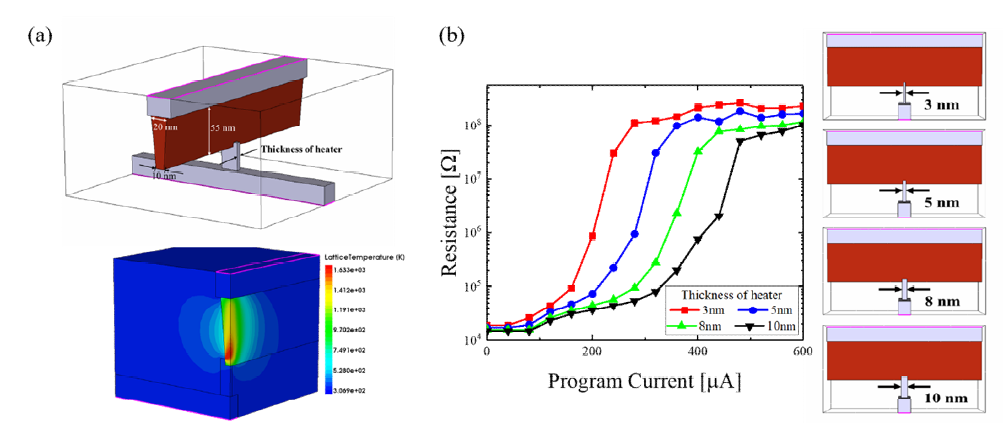One can see that the reset transition in the line-andspace pattern occurs at much higher current than the confined patterns. There is the trade-off between the gradualness and the reset current. In our simulations, higher gradualness was obtained by higher heat dissipation that in turn degrades the reset current. It is expected that the necessary gradualness cannot be achieved just by simple structure engineering. One needs to find a mitigation point or an innovative new architecture.

### 2. Phase-change Material Engineering

The standard phase-change material is currently the GST, Ge2Sb2Te5 whose standard properties are listed in Table 1. In practice, each chip maker that is developing the PC-RAM has its own recipé for GST, for example, compositional variation, different doping elements, etc. Such material engineering yields a significant change in material properties[25-28]. It is not yet clear which combination of dopants and composition is the most effective for synapse devices. What can be done by simulation is a kind of reverse engineering that can guide a direction of modifying material properties. Hereafter, we will refer a phase-change material to GST because the term, GST, is also used for indicating a general phasechange material in the PC-RAM society. We selected two properties of the GST for our investigation, resistivity and thermal conductivity. Both are for the crystalline state. We are simulating the crystal-to-amorphous transition. Thus, the crystalline properties are important during temperature rising.

The simulated transition curves for reducing resistivity by two orders are presented in Fig. 5. In case of the confined pattern as shown in Fig. 5(a), the reset current increases about seven times for decreasing the resistivity by two orders. Compared to the huge loss in the reset current, the improvement in the gradualness is minute. In case of the line-and-space pattern as shown in Fig. 5(b), the reset current also increases about seven times for the same resistivity change with the confined case. However, the improvement in the gradualness is clearer.

Fig. 5. The reset transition curves for different resistivity (a) the confined pattern, (b) the line and space pattern.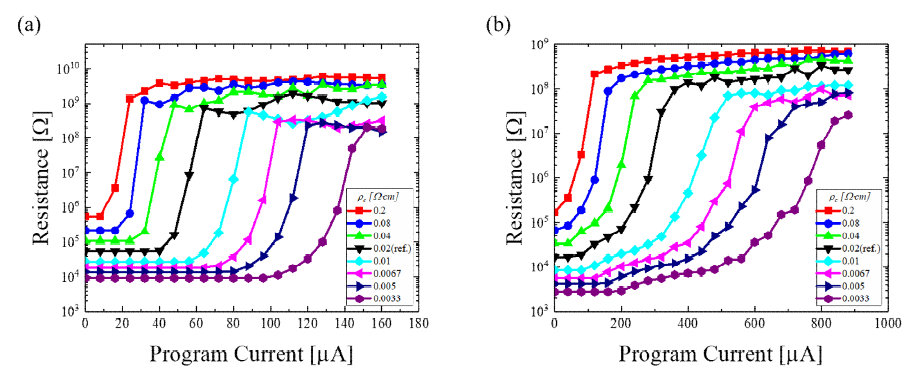Note that there are some fluctuations in resistance after achieving the full reset in case of the confined pattern shown in Fig. 5(a). This is the overheating and recrystallization effect that is enhanced by four-sided TBRs. One cannot see such fluctuation in case of the line-and-space GST in which heat flows out well.

The results for reducing thermal conductivity by onetwentieth are presented in Fig. 6. The range of the thermal conductivity is narrower than that of the resistivity, around one-hundredth. In fact, the thermal conductivity composed of phonon and electronic/hole parts is more immune to doping than the resistivity with no phonon contribution is. Doping mostly contributes to carrier concentration and does not affect the phonon part of the thermal conductivity. Reducing thermal conductivity also results in higher gradualness in the reset transition curve.

Fig. 6. The reset transition curves for different thermal conductivity (a) the confined pattern, (b) the line and space pattern.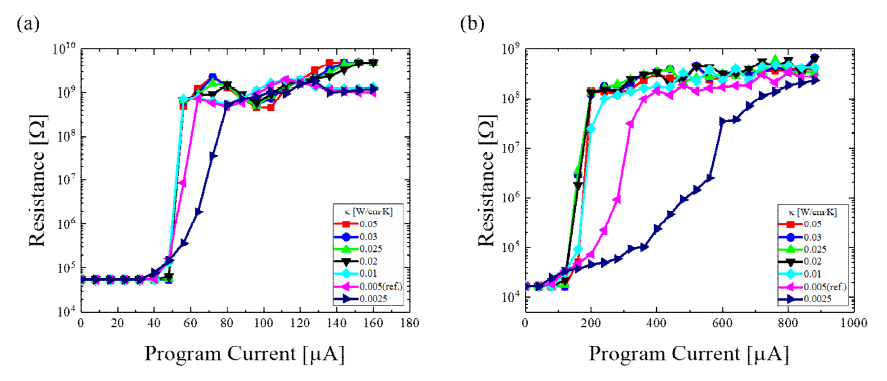For the low current, the smaller thermal conductivity lets the heat generated by Joule heating more concentrated in the smaller area, which leads to the onset current reduction. For the high current, the smaller thermal conductivity prevents the generated heat from traveling far and thus it becomes more difficult to make a larger melt region which is subject to become an amorphous region. The more difficulty in making a larger amorphous region leads to more gradualness because the resistance mostly depends upon the size of the amorphous region.

Overall, the line-and-space pattern shows much higher gradualness than the confined pattern does due to the roles of the TBRs. In terms of material engineering, decreasing both resistivity and thermal conductivity yields higher gradualness. These days, material engineering to optimize memory characteristics are actively being investigated by an innovative way using computational and analytical tools[29,30]. Such works for synapse characteristics should be performed.

## IV. CONCLUSIONS

The gradualness of the reset transition in the PC-RAM was investigated using TCAD simulation. For the device structural aspect, a comparative study between the confined and the line-and-space GST patterns revealed that the former has advantage in the reset current but shows an abrupt transition, and that the latter is vice versa. For the material aspect, varying the resistivity and the thermal conductivity of the GST also yielded the trade-off between the reset current and the gradualness. Overcoming this trade-off by designing an innovative cell scheme should be a key factor to develop a phasechange synapse with both low power and high gradualness.

### ACKNOWLEDGMENTS

This research was supported by Nano Material Technology Development Program through the National Research Foundation of Korea (NRF) funded by the Ministry of Science, ICT and Future Planning (NRF-2016M3A7B4910398), by Basic Science Research Program through the National Research Foundation of Korea (NRF) funded by the Ministry of Education (2018R1D1A1B05050256), by 2018 Hongik University Research Fund, and by the IC Design Education Center through the EDA Tool.

### REFERENCES

1
Indiveri G., et al , May 2011, 제목, Front. Neurosci, Vol. 5, pp. 732
Poon C. S., Zhou K., Sep. 2011, Neuromorphic silicon neurons and large-scale neural networks: challenges and opportunities, Front. Neurosci, Vol. 5, pp. 1083
Kang D. H., et al , May 2015, Emulation of spike-timing dependent plasticity in nano-scale phase change memory, Neurocomputing, Vol. 155, pp. 153-1584
Suri M., et al , Dec. 2011, Phase change memory as synapse for ultra-dense neuromorphic systems: Application to complex visual pattern extraction, Proc. IEEE IEDM, pp. 4.4.1-4.4.45
Suri M., et al , Sep. 2012, Physical aspects of low power synapses based on phase change memory devices, J. Appl. Phys., Vol. 112, No. 56
Burr G. W., et al , Nov. 2015, Experimental demonstration and tolerancing of a large-scale neural network (165000 synapses) using phase-change memory as the synaptic weight element, IEEE Trans. Electron Devices, Vol. 62, No. 11, pp. 3498-35077
Kuzum D., et al , Jun. 2011, Nanoelectronic programmable synapses based on phase change materials for brain-inspired computing, Nano Lett., Vol. 12, No. 5, pp. 2179-21868
Ambrogio S., et al , Mar. 2016, Unsupervised learning by spike timing dependent plasticity in phase change memory (PCM) synapses, Front. Neurosci., Vol. 10, pp. 56-679
Wong H. S., et al , Dec. 2010, Phase change memory, Proc. IEEE, Vol. 98, No. 12, pp. 2201-222710
Im D. H., et al , Dec. 2008, A unified 7.5 nm dash-type confined cell for high performance PRAM device, Proc. IEEE IEDM, pp. 1-411
Schmithusen B., Tikhomirov P., Lyumkis E., Sep. 2008, Phase-change memory simulations using an analytical phase space model, Proc. Int. Conf. Simul. Semicond. Process. Devices (SISPAD), pp. 57-6012
Avrami M., Dec. 1939, Kinetics of phase change. I. General theory, J. Chem. Phys., Vol. 7, No. 12, pp. 1103-111213
Peng C., Cheng L., Mansuripur M., Sep. 1997, Experimental and theoretical investigations of laser-induced crystallization and amorphization in phase-change optical recording media, J. Appl. Phys., Vol. 82, No. 9, pp. 4183-419114
Senkadar S., Wright C. D., Feb. 2004, Models for phasechange of Ge2Sb2Te5 in optical and electrical memory devices, J. Appl. Phys., Vol. 95, No. 2, pp. 504-51115
Servalli G., Dec. 2009, A 45nm generation phase change memory technology, Proc. IEEE IEDM, pp. 1-416
Boniardi M., et al , Dec. 2014, Optimization metrics for phase change memory (PCM) cell architectures, Proc. IEEE IEDM, pp. 29.1.1-29.1.417
Reifenberg J. P., et al , Oct. 2008, The impact of thermal boundary resistance in phase-change memory devices, IEEE Electron Device Lett., Vol. 29, No. 10, pp. 1112-111418
Kencke D. L., et al , Dec. 2007, The role of interfaces in damascene phase-change memory, Proc. IEEE IEDM, pp. 323-32619
Kim E. K., et al , Jun. 2000, Thermal boundary resistance at Ge2Sb2Te5/ZnS:SiO2 interface, Appl. Phys. Lett., Vol. 76, No. 26, pp. 3864-386620
Pirovano A., et al , Mar. 2004, Electronic switching in phasechange memories, IEEE Trans. Electron Devices, Vol. 51, No. 321
Ferrari G., et al , Sep. 2010, Multiphysics modeling of PCM devices for scaling investigation, Proc. Int. Conf. SISPAD, pp. 265-26822
Konstantinov P. P., et al , Jul. 2001, Thermoelectric properties of nGeTe center dot mSb2Te3 layered compounds, Inorganic Mater., Vol. 37, No. 7, pp. 662-66823
Kim D. H., et al , Mar. 2007, Three-dimensional simulation model of switching dynamics in phase change random access memory cells, J. Appl. Phys., Vol. 101, No. 624
Khulbe P. K., et al , Oct. 2000, Crystallization behavior of asdeposited, melt quenched, and primed amorphous states of Ge2Sb2.3Te5 films, J. Appl. Phys., Vol. 88, No. 7, pp. 3926-393325
Raoux S., et al , Nov. 2007, Direct observation of amorphous to crystalline phase transitions in nanoparticle arrays of phase change materials, J. Appl. Phys., Vol. 102, No. 9, pp. 09430526
Horii H., et al , Jun. 2003, A novel cell technology using Ndoped GeSbTe films for phase change RAM, Proc. Symp. VLSI Tech. Dig., pp. 177-17827
Qiao B., et al , Oct. 2006, Effects of Si doping on the structural and electrical properties of Ge2Sb2Te5 films for phase change random access memory, Appl. Surf. Sci., Vol. 252, No. 24, pp. 8404-840928
Zhou X., et al , Oct. 2012, Carbon-doped Ge2Sb2Te5 phase change material: A candidate for high-density phase change memory application, Appl. Phys. Lett., Vol. 101, No. 14, pp. 14210429
Akola J., Jones R. O., Dec. 2017, Speeding up crystallization, Science, Vol. 358, No. 6369, pp. 1386-138630
Rao F., et al , 2017, Reducing the stochasticity of crystal nucleation to enable subnanosecond memory writing, Science, Vol. 358, pp. 1423-1427## Author

was born in Boryeongsi, Chungcheongnam-do, Korea, in 1991.

He received B.S. and M.S. in materials science and engineering from Hongik University, Seoul, Korea, in 2017 and 2019, respectively.

His thesis research was device modeling of phase-change memory using phasefield method.

was born in Uijeongbu-si, Gyeonggi-do, Korea, in 1991.

He received B.S. and M.S. in materials science and engineering from Hongik University, Seoul, Korea, in 2017 and 2019, respectively.

His thesis research was a macroscopic and stochastic model for switching behavior in resistive memory using finite element method.

was born in Bucheonsi, Gyeonggi-do, Korea, in 1993.

She received B.S. and M.S. in materials science and engineering from Hongik University, Seoul, Korea, in 2016 and 2018, respectively.

Her thesis research was theoretical study on organic-inorganic hybrid perovskite/metal electrode contacts.

was born in Seoul, South Korea, in 1974.

He received B.S. in Materials Science and Engineering from Seoul National University, Korea in 2000.

Then, he received Ph. D in Materials Science and Engineering from Northwestern University, USA in 2007.

He worked at Semiconductor R & D Center, Samsung Electronics from Aug. 2007 to Feb. 2013 and participated in the development of 82, 58, 39, 20 nm phase-change random access memories (PRAMs).

He joined the Department of Materials Science and Engineering, Hongik University as a faculty member in Mar. 2013.

His main research is the computational modeling of the microstructure evolution in materials.

The switching mechanisms of phase-change memory and memristors fall into this category.

Other topics are coarsening of bicontinuous mixtures, morphological evolution of nanoparticles, grain growth in polycrystalline materials, etc.